# Java温习——数组

## 2 定义

<数组元素的数据类型>[] <数组名>; // 定义方式1，如int[] ages;
<数组元素的数据类型> <数组名>[]; // 定义方式2，如int ages[];

## 3 数组的初始化

### （1）静态初始化

a 概念

<数组元素的数据类型>[] <数组名> = new <数组元素的数据类型>[]{元素1, 元素2, 元素3, ...}; // 如int[] ages = new int[]{17, 18, 19};
<数组元素的数据类型>[] <数组名> = {元素1, 元素2, 元素3, ...}; // 简单形式的初始化

b 内存分析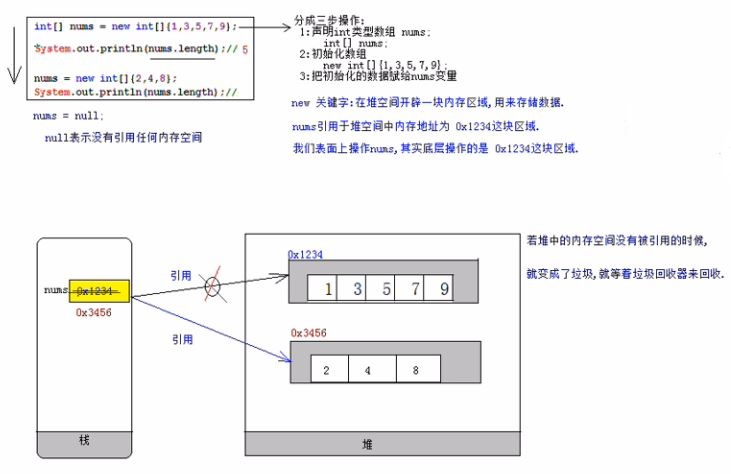### （2）动态初始化

a 概念

int <数组元素的数据类型>[] <数组名> = new <数组元素的数据类型>[<int类型的数组长度>]; // 如int[] ages = new int;


b 不同数据类型的初始值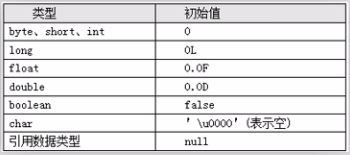c 内存分析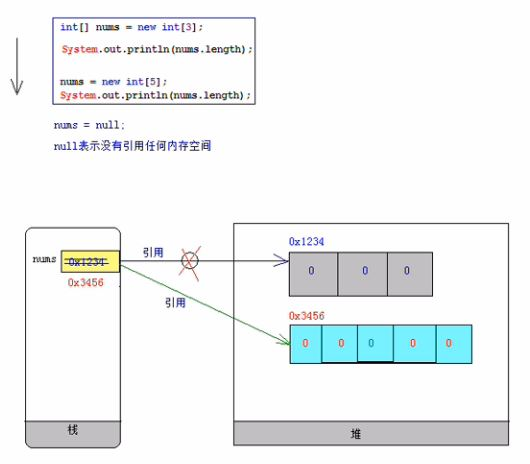## 4 数组基本操作

### （1）获取元素

<数组元素的数据类型> <变量名> = <数组名>[<索引index>];

### （2）设置元素

<数组名>[<index>] = <数值>;

### （3）遍历元素

int[] ages = new int[]{1,2,3};
for(int index = 0; index < ages.length; index ++){
System.out.println(ages[index]);
}


### （4）获取数组长度

int len = <数组名>.length // length是属性，而非方法

### （5）获取索引范围

[ 0, <数组名>.length-1]

## 6 数组编程

### （1）获取数组最大/小元素

static int maxValue(int num[]){
if( num == null || num.length == 0){ // 首先判断传递过来的数组是否为空，或者数组长度为0，若是则直接返回，否则才进行后续操作
return -1;
}
int len = num.length;
int max = num;
for(int i = 1; i < len; i ++){
if(max < num[i]){
max = num[i];
}
}
return max;
}

static int minValue(int num[]){
if( num == null || num.length == 0){
return -1;
}
int len = num.length;
int min = num;
for(int i = 1; i < len; i ++){
if(min > num[i]){
min = num[i];
}
}
return min;
}

### （2）按格式打印数组元素

static String printArray(int[] num){
if( num == null || num.length == 0){
return "[]";
}
int len = num.length;
String str = "[";
for(int i = 0; i < len; i++){
str += num[i];
if(i != len - 1){
str += ", ";
}
}
str += "]";
return str;
}

### （3）逆序排列数组

static void reverseArray(int[] num){
if(num == null || num.length == 0){
return;
}
int len = num.length;
int halfLen = num.length / 2;
int temp;
for(int i = 0; i < halfLen; i++){
temp = num[i];
num[i] = num[len - 1 - i];
num[len - 1 - i] = temp;
}
return;
}


### （4）元素第一次/最后一次出现索引（线性搜索）

static int firstIndex(int[] arr, int num){
if(arr == null || arr.length == 0){
return -1;
}
int len = arr.length;
for(int i = 0; i < len; i++){
if(num == arr[i]){
return i;
}
}
return -1;
}
static int lastIndex(int[] arr, int num){
if(arr == null || arr.length == 0){
return -1;
}
int len = arr.length;
for(int i = len - 1; i >= 0; i--){
if(arr[i] == num){
return i;
}
}
return -1;
}

## 7 方法参数的值传递机制

### （1）main方法的数组参数

main方法是static修饰的，可直接使用所在类的类名进行调用；

main方法的String数组参数，其实是暴露给程序运行者，用于给程序传递一个数据信息——任何程序交互的窗口；

args表示第一个参数，以此类推；

class Hello
{
public static void main(String[] args)
{
System.out.println(args.length);
for(int i = 0; i < args.length; i ++){
System.out.print(args[i] + "\t");
}
System.out.println();
}
}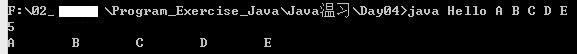### （2）基本数据类型

class ParameterDemo
{
static void change(int x){
System.out.println("change方法修改前: " + x); // 10
x = 50;
System.out.println("change方法修改后: " + x); // 50
}
public static void main(String[] args)
{
int x = 10;
System.out.println("main方法修改前: " + x); // 10
ParameterDemo.change(x); // 改变x变量
System.out.println("main方法修改后: " + x); // 10，这里易出错
}
}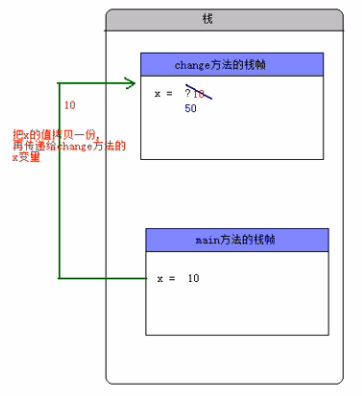### （3）引用数据类型

class ParameterDemo
{
static void printArray(int[] arr){
if(arr == null || arr.length == 0){
return;
}
String str = "[";
for(int i = 0; i < arr.length; i++){
str += arr[i];
if(i != arr.length - 1){
str += ", ";
}
}
str += "]";
System.out.println(str);
}
static void swap(int[] arr){
System.out.print("change方法修改前: "); // [10, 99]
ParameterDemo.printArray(arr);
int temp;
temp = arr;
arr = arr;
arr = temp;
System.out.print("change方法修改后: "); // [99, 10]
ParameterDemo.printArray(arr);
}
public static void main(String[] args)
{
int[] arr = new int[]{10, 99};
System.out.print("main方法修改后: "); // [10, 99]
ParameterDemo.printArray(arr);
ParameterDemo.swap(arr);
System.out.print("main方法修改后: "); // [99, 10]
ParameterDemo.printArray(arr);
}
}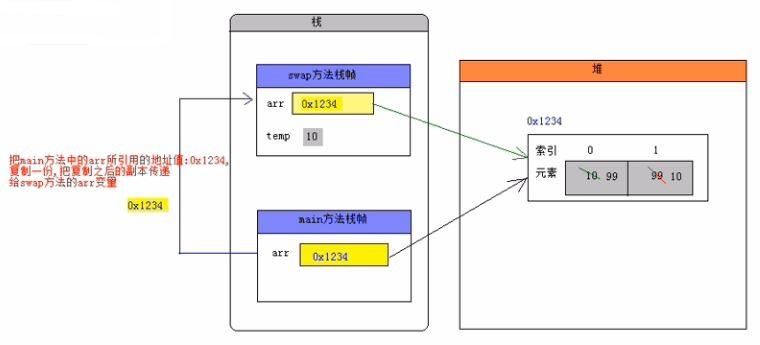## 8 多维数组

### （1）二维数组概念

int[][] arr = {
{1, 2, 3},
{4, 5},
{6}
};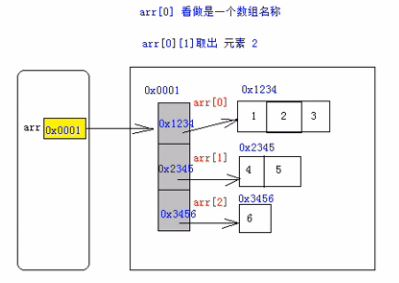### （2）二维数组的操作

a 初始化操作

int[][] <二维数组名> = new int [][]{一维数组1, 一维数组2, 一维数组3, ...};
int[][] <二维数组名> = {一维数组1, 一维数组2, 一维数组3, ...};

int[][] <二维数组名> = new int[<行数m>][<列数n>]; // 创建一个长度为m的二维数组，其中每个元素（一维数组）的长度是n

b 迭代操作

for(int i = 0; i < arr1.length; i ++){
for(int j = 0; j < arr1[i].length; j++){
// 相应操作
}
}


## 9 Java5对数组的新语法支持

### （1）增强for循环 —— foreach

for(<数组元素的数据类型> <变量> : <数组名>){
// 相应操作
}

int[] nums = {10, 20, 30, 40, 50};
for(int num : nums){
System.out.println(num);
}


foreach在底层依然使用for循环+索引操作数组，因此把增强for循环称为编译器的新特性——语法糖（让程序员写更少、更简单的代码完成相同的功能）；

for循环比foreach循环功能更强大；

### （2）方法的可变参数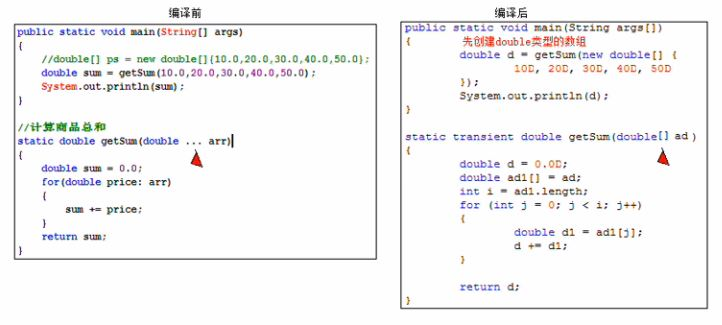## 10 数组算法

### （1）实现int类型数组元素拷贝

class ParameterDemo
{
static void printArray(int[] arr){
if(arr == null || arr.length == 0){
return;
}
for(int i = 0; i < arr.length; i ++){
System.out.print(arr[i]);
if(i != arr.length - 1){
System.out.print("\t");
}
}
System.out.println();
}
static void copy(int[] src, int[] dest, int srcPos, int destPos, int length){
for(int i = srcPos, j = destPos; i < srcPos + length; i ++, j ++){
dest[j] = src[i];
}
}
public static void main(String[] args)
{
int[] src = new int[]{1,2,3,4,5,6,7,8,9,10};
int[] dest = new int;
ParameterDemo.printArray(dest);
ParameterDemo.copy(src, dest, 2, 5, 4);
ParameterDemo.printArray(dest);
}
}


### （2）System类中的arraycopy方法

public static native void arraycopy(Object src, int srcPos, Object dest, int destPos, int length);

class ArrayCopyDemo
{
static void printArray(int[] arr){
if(arr == null || arr.length == 0){
return;
}
for(int i = 0; i < arr.length; i ++){
System.out.print(arr[i]);
if(i != arr.length - 1){
System.out.print("\t");
}
}
System.out.println();
}
public static void main(String[] args)
{
int[] src = {1,2,3,4,5,6,7,8,9,10};
int[] dest = new int;
ArrayCopyDemo.printArray(dest);
System.arraycopy(src, 2, dest, 5, 4);
ArrayCopyDemo.printArray(dest);
}
}


### （3）排序算法 —— 冒泡算法

a 概念

b 分类

class BubbleSortDemo {
static void printArray(int[] arr){
if(arr == null || arr.length == 0){
return;
}
for(int i = 0; i < arr.length; i ++){
System.out.print(arr[i]);
if(i != arr.length - 1){
System.out.print("\t");
}
}
System.out.println();
}
static void swap(int[] arr, int i, int j){
int temp;
temp = arr[i];
arr[i] = arr[j];
arr[j] = temp;
}
static void bubbleSort(int[] arr){
if(arr == null || arr.length == 0){
return;
}
int temp;
for(int i = 0; i < arr.length - 1; i ++){
for(int j = 1; j < arr.length - i; j ++){
if(arr[j - 1] > arr[j]){
BubbleSortDemo.swap(arr, j - 1, j);
}
}
}
}
public static void main(String[] args) {
int[] arr = {2, 9, 6, 7, 4, 1};
BubbleSortDemo.printArray(arr);
BubbleSortDemo.bubbleSort(arr);
BubbleSortDemo.printArray(arr);
}
}


class SelectSortDemo
{
static void printArray(int[] arr){
if(arr == null || arr.length == 0){
return;
}
for(int i = 0; i < arr.length; i ++){
System.out.print(arr[i]);
if(i != arr.length - 1){
System.out.print("\t");
}
}
System.out.println();
}
static void swap(int[] arr, int i, int j){
int temp;
temp = arr[i];
arr[i] = arr[j];
arr[j] = temp;
}
static void selcetSort(int[] arr){
if(arr == null || arr.length == 0){
return;
}
for(int i = 0; i < arr.length - 1; i ++){
for(int j = i + 1; j < arr.length; j ++){
if(arr[i] > arr[j]){
SelectSortDemo.swap(arr, i, j);
}
}
}
}
public static void main(String[] args)
{
int[] arr = {2, 9, 6, 7, 4, 1};
SelectSortDemo.printArray(arr);
SelectSortDemo.selcetSort(arr);
SelectSortDemo.printArray(arr);
}
}


### （4）搜索算法

a 概念

b 分类

class MidFindDemo
{
static void printArray(int[] arr){
if(arr == null || arr.length == 0){
return;
}
for(int i = 0; i < arr.length; i ++){
System.out.print(arr[i]);
if(i != arr.length - 1){
System.out.print("\t");
}
}
System.out.println();
}
static int midFind(int[] arr, int num){
if(arr == null || arr.length == 0){
return -1;
}
int low = 0;
int high = arr.length - 1;
int mid = (low + high) / 2;
while(low <= high){
if(arr[mid] < num){
low = mid + 1;
}else if(arr[mid] > num){
high = mid - 1;
}else{
return mid;
}
mid =  (low + high) / 2;
}
return -1;
}
public static void main(String[] args)
{
int[] arr = {1, 2, 4, 6, 7, 9};
int num = 4;
MidFindDemo.printArray(arr);
System.out.println(num);
System.out.println(MidFindDemo.midFind(arr, num));
}
}


### （6）Java内置数组工具类Arrays

int binarySearch(type[] arr, type key) —— 使用二分法查找数组中某元素并返回其索引，若找不到则返回负数

void sort(type[] arr) —— 使用调优后的快速法对指定数组排序

String toString(type[] arr) —— 返回指定数组内容的字符串表示形式

public static type[] copyOf(type[] original, int newLength) —— 复制指定数组，截取或用0补充（如有必要），以使副本具有指定长度

©️2019 CSDN 皮肤主题: 编程工作室 设计师: CSDN官方博客